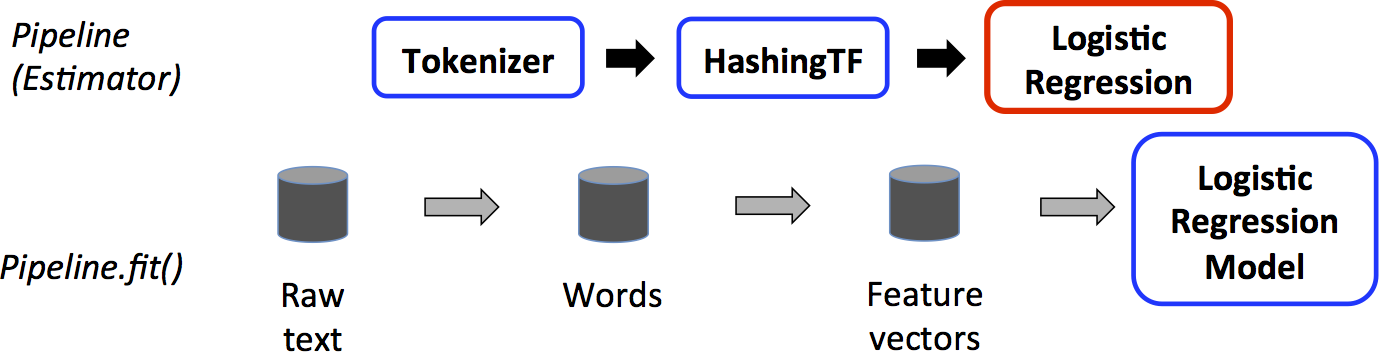﻿ Introduction to Spark’s Machine Learning Pipeline – BMC Blogs
Machine Learning & Big Data Blog

# Introduction to Spark’s Machine Learning PipelinebyHere we explain what is a Spark machine learning pipeline. We will do this by converting existing code that we wrote, which is done in stages, to pipeline format. This will run all the data transformation and model fit operations under the pipeline mechanism.

The existing Apache Spark ML code is explained in two blog posts: part one and part two. You are encouraged to read that first as you will need to do that to generate data to feed into this program. Plus you will understand what we have changed and thus learn the pipeline concept. (Or if you want to take a shortcut and skip reading that you could just use the maintenance_data.csv as both the test and training data.)

The Spark pipeline object is org.apache.spark.ml.{Pipeline, PipelineModel}.

In general a machine learning pipeline describes the process of writing code, releasing it to production, doing data extractions, creating training models, and tuning the algorithm. It should be a continuous process as a team works on their ML platform. But for Apache Spark a pipeline is an object that puts transform, evaluate, and fit steps into one object org.apache.spark.ml.Pipeline. These steps are called a workflow. (Presumably there are some performation, distribution. or other benefits to doing this, but the Spark documentation does not spell that out.) But at least it mimics the pipeline from, at least regarding the data transformation operations.

To start, we look at the graphic supplied by Apache Spark. Basically you start with creating a dataframe then you put any transformation steps into the pipeline object plus the ML algorithm you will use.In other words, in the graphic above the dataframe is created through reading data from Hadoop or whatever and then transform() and fit() operations are performed on it to add feature and label columns, which is the format required for the logistic regression ML algorithm. The discrete several steps are fed into the pipeline object. Transform means to modify a dataframe, such as adding features and labels columns. Fit means to feed the dataframe into the ML algorithm and then calculate the answer, i.e. create the model. You can also run transform directly on a dataframe. In a sense this is what the pipeline does for us.

With regards to the graphic above, the code shown below shows how that is implemented. Each of these three steps will be handled by the pipeline object in: val pipeline = new Pipeline().setStages(Array(tokenizer, hashingTF, lr)).

```val tokenizer = new Tokenizer()
.setInputCol("text")
.setOutputCol("words")
val hashingTF = new HashingTF()
.setNumFeatures(1000)
.setInputCol(tokenizer.getOutputCol)
.setOutputCol("features")
val lr = new LogisticRegression()
.setMaxIter(10)
.setRegParam(0.001)
val pipeline = new Pipeline()
.setStages(Array(tokenizer, hashingTF, lr))```

To illustrate using our own code, we rewrite the code from the blog posts mentioned above, which was two separate programs (create model and make predictions) into one program shown here.

First we have the usual imports.

```import org.apache.spark.sql.SQLContext
import org.apache.spark.SparkConf
import org.apache.spark.SparkContext
import com.databricks.spark.csv
import org.apache.spark.ml.classification.LogisticRegression
import org.apache.spark.mllib.linalg.{Vector, Vectors}
import org.apache.spark.ml.evaluation.BinaryClassificationEvaluator
import org.apache.spark.ml.feature.{VectorAssembler, StringIndexer}
import org.apache.spark.ml.classification.LogisticRegressionModel
import org.apache.spark.ml.{Pipeline, PipelineModel}
import org.apache.spark.sql.Row```

Next, we first read in the dataframe from a text file as usual but instead of performing transform() operations by themselves on the dataframe, we feed the VectorAssembler(), StringIndexer(), and LogisticRegression() into new Pipeline().setStages(Array(assembler, labelIndexer, lr)). Then we run pipeline.fit() on the original dataframe. The pipeline knows what transformations to run and in which order because we specified that here .setStages(Array(assembler, labelIndexer, lr)). At the end we have our trained model.

Again, here is the old code. After that is the new.

```val df2 = assembler.transform(df)

val df3 = labelIndexer.fit(df2).transform(df2)

val model = new LogisticRegression().fit(df3)```

New code:

```var file = "hdfs://localhost:9000/maintenance/maintenance_data.csv";

val sqlContext = new SQLContext(sc)

val featureCols =  Array("lifetime", "pressureInd", "moistureInd", "temperatureInd")

val assembler = new VectorAssembler().setInputCols(featureCols).setOutputCol("features")

val labelIndexer = new StringIndexer().setInputCol("broken").setOutputCol("label")

val lr = new LogisticRegression()

val pipeline = new Pipeline().setStages(Array(assembler, labelIndexer, lr))

val model = pipeline.fit(df)```

Now the predictions are easy. The model already knows what transformations to run. So we just read in the test data (You created that in blog post part one.) and run transform() on it. Then we filter those whose logistic regression value is > 0, i.e., 1, the print out those machines that require maintenance.

```var predictFile = "hdfs://localhost:9000/maintenance/2018.05.30.09.46.55.csv"

val predictions =  model.transform(testdf)

predictions.select("team", "provider", "prediction").filter(\$"prediction" > 0).collect().foreach { case Row(team: String,provider: String, prediction: Double) =>
println(s"(\$team, \$provider, \$prediction) --> team=\$team, provider=\$provider, prediction=\$prediction")
}```

The results look like this:

```(TeamA, Provider4, 1.0) --> team=TeamA, provider=Provider4, prediction=1.0
(TeamC, Provider2, 1.0) --> team=TeamC, provider=Provider2, prediction=1.0
(TeamC, Provider4, 1.0) --> team=TeamC, provider=Provider4, prediction=1.0
(TeamB, Provider1, 1.0) --> team=TeamB, provider=Provider1, prediction=1.0
(TeamC, Provider2, 1.0) --> team=TeamC, provider=Provider2, prediction=1.0
(TeamB, Provider2, 1.0) --> team=TeamB, provider=Provider2, prediction=1.0
(TeamA, Provider2, 1.0) --> team=TeamA, provider=Provider2, prediction=1.0```

### Wikibon: Automate your Big Data pipeline

Learn how data management experts throughout the industry are transforming their Big Data infrastructure for maximum business impact.

These postings are my own and do not necessarily represent BMC's position, strategies, or opinion.

See an error or have a suggestion? Please let us know by emailing blogs@bmc.com.

### About the author#### Walker Rowe

Walker Rowe is an American freelance tech writer and programmer living in Tunisia. He specializes in big data, analytics, and programming languages. Find him on LinkedIn or Upwork.